# Homology product

(diff) ← Older revision | Latest revision (diff) | Newer revision → (diff)

An operation defined on the groups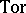and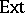. One considers-algebrasand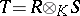over a commutative ring. The derived functors (cf. Derived functor)andover them may be combined with one another by means of four homomorphisms, known as homology products: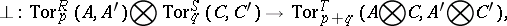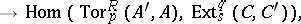Here,and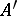are right or left-modules,and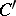are right or left-modules, while the symbolis omitted in all functors. The last two homomorphisms are defined only if the algebrasandare projective overand if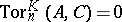for all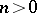. If certain supplementary restrictions are made, intrinsic products can be obtained which connectandover the same ring.

All four products can be obtained from formulas representing the functors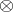and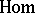by replacing the arguments by the corresponding resolutions . The multiplication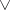permits the following interpretation in terms of Yoneda products. Let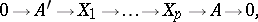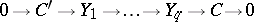be exact sequences of- and-modules, respectively, that are representatives of the corresponding equivalence classes inand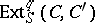. Multiplying the former tensorially from the right byand the latter from the left by, one obtains exact sequences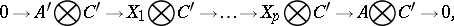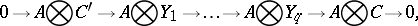which can be combined into the exact sequence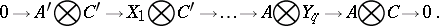This sequence can be regarded as the representative of an equivalence class in the group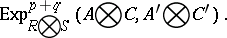The productin the cohomology space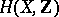of a topological spacewith coefficients in the ring of integers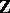is known as the Alexander–Kolmogorov product or the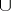-product.

How to Cite This Entry:
Homology product. Encyclopedia of Mathematics. URL: http://encyclopediaofmath.org/index.php?title=Homology_product&oldid=18757
This article was adapted from an original article by V.E. Govorov (originator), which appeared in Encyclopedia of Mathematics - ISBN 1402006098. See original article